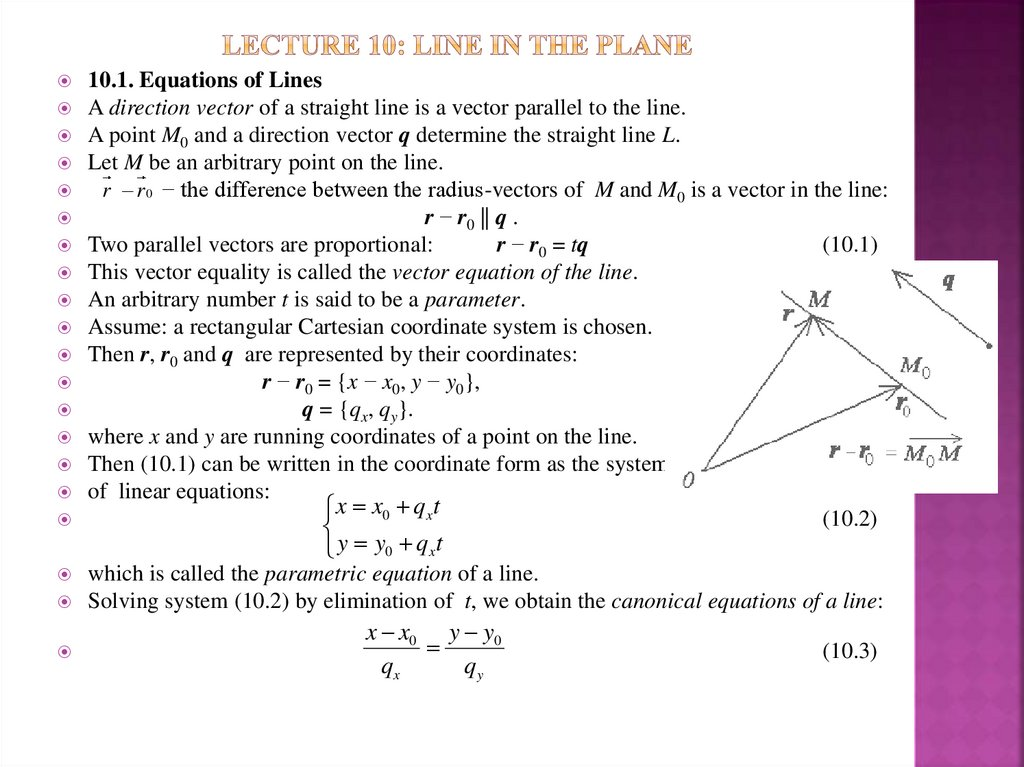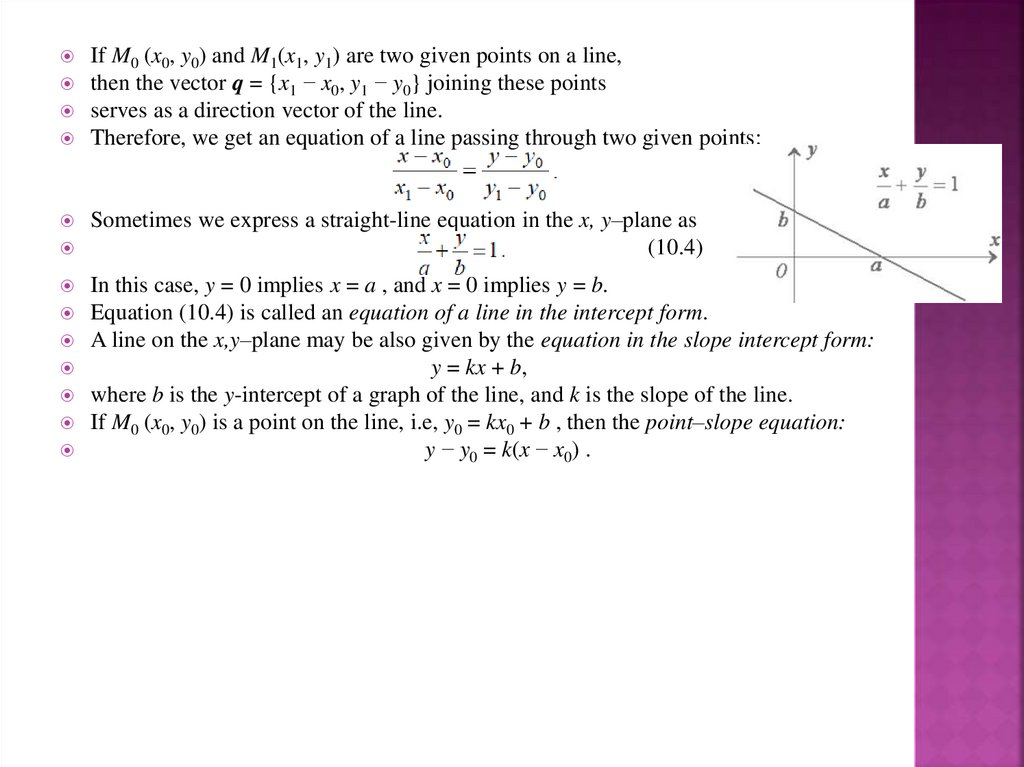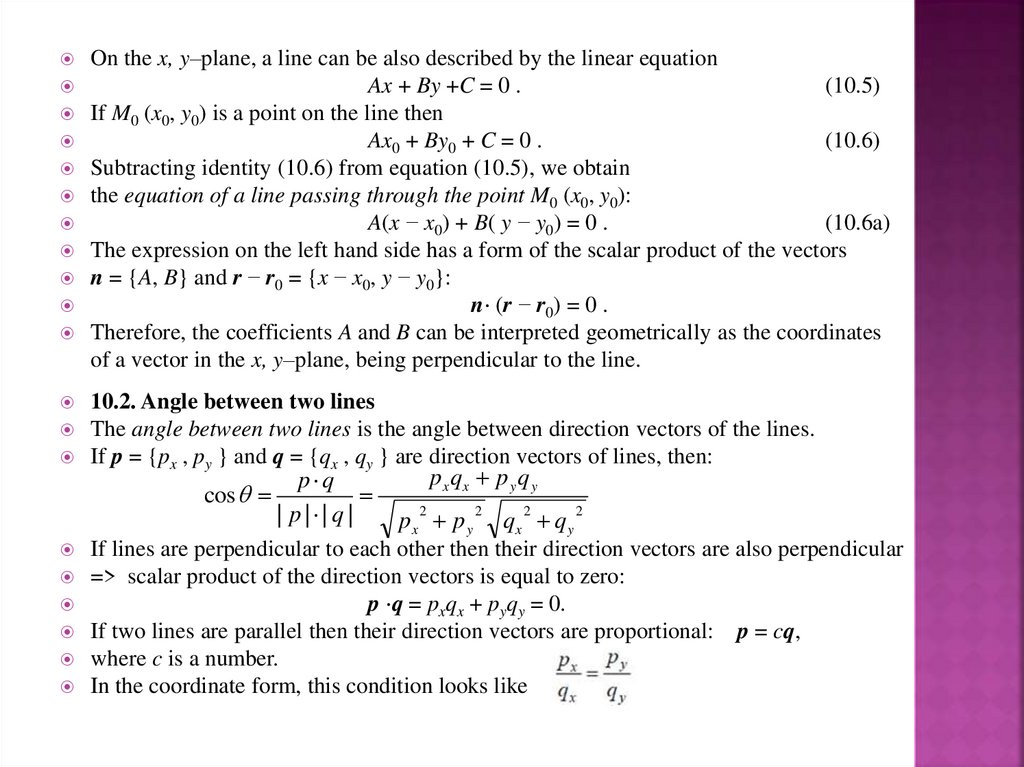# Line in the Plane. Lecture 10

## 1. Lecture 10: Line in the Plane

10.1. Equations of Lines
A direction vector of a straight line is a vector parallel to the line.
A point M0 and a direction vector q determine the straight line L.
Let M be an arbitrary point on the line.
r r 0 − the difference between the radius-vectors of M and M0 is a vector in the line:
r − r0 || q .
Two parallel vectors are proportional:
r − r0 = tq
(10.1)
This vector equality is called the vector equation of the line.
An arbitrary number t is said to be a parameter.
Assume: a rectangular Cartesian coordinate system is chosen.
Then r, r0 and q are represented by their coordinates:
r − r0 = {x − x0, y − y0},
q = {qx, qy}.
where x and y are running coordinates of a point on the line.
Then (10.1) can be written in the coordinate form as the system
of linear equations:
x x0 q x t
(10.2)
y y0 q x t
which is called the parametric equation of a line.
Solving system (10.2) by elimination of t, we obtain the canonical equations of a line:
x x0 y y0
(10.3)
qx
qy

## 2.

If M0 (x0, y0) and M1(x1, y1) are two given points on a line,
then the vector q = {x1 − x0, y1 − y0} joining these points
serves as a direction vector of the line.
Therefore, we get an equation of a line passing through two given points:
Sometimes we express a straight-line equation in the x, y–plane as
(10.4)
In this case, y = 0 implies x = a , and x = 0 implies y = b.
Equation (10.4) is called an equation of a line in the intercept form.
A line on the x,y–plane may be also given by the equation in the slope intercept form:
y = kx + b,
where b is the y-intercept of a graph of the line, and k is the slope of the line.
If M0 (x0, y0) is a point on the line, i.e, y0 = kx0 + b , then the point–slope equation:
y − y0 = k(x − x0) .

## 3.

On the x, y–plane, a line can be also described by the linear equation
Ax + By +C = 0 .
(10.5)
If M0 (x0, y0) is a point on the line then
Ax0 + By0 + C = 0 .
(10.6)
Subtracting identity (10.6) from equation (10.5), we obtain
the equation of a line passing through the point M0 (x0, y0):
A(x − x0) + B( y − y0) = 0 .
(10.6a)
The expression on the left hand side has a form of the scalar product of the vectors
n = {A, B} and r − r0 = {x − x0, y − y0}:
n⋅ (r − r0) = 0 .
Therefore, the coefficients A and B can be interpreted geometrically as the coordinates
of a vector in the x, y–plane, being perpendicular to the line.
10.2. Angle between two lines
The angle between two lines is the angle between direction vectors of the lines.
If p = {px , py } and q = {qx , qy } are direction vectors of lines, then:
px qx p y q y
p q
cos
2
2
2
2
| p | | q |
px p y qx q y
If lines are perpendicular to each other then their direction vectors are also perpendicular
=> scalar product of the direction vectors is equal to zero:
p ⋅q = pxqx + pyqy = 0.
If two lines are parallel then their direction vectors are proportional: p = cq,
where c is a number.
In the coordinate form, this condition looks like

## 4.

If two lines in the x, y–plane are given by the equations in the slope intercept form
y = k1x + b1 and y = k2x + b2,
and θ is the angle between the lines, then
The lines are parallel, if
The lines are perpendicular, if
k1 = k2.
k1k2 = −1.
10.3. Distance From a Point to a Line
Consider a line in the x, y–plane.
Let n be a normal vector to the line and M(x0, y0) be any point on the line.
Then the distance d from a point P not on the line is equal to the absolute value of the
projection of the vector PM on n:
In particular, if the line is given by the equation Ax + By +C = 0 ,
and the coordinates of the point P are x1 and y1, that is,
n = {A, B} and PM = {x1 − x0, y1 − y0},
then the distance from P to the line is calculated according to the following formula:
Since M(x0, y0) is a point on the line, Ax0 + By0 + C = 0 .
Therefore, we obtain